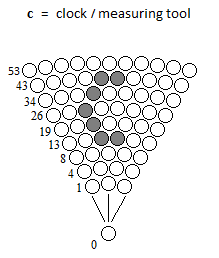# Consequence State

## Paradox and Stop Motion

With the anharmonic oscillator, the repeated high frequency alternation of the particle can give the appearance of static state of the mass between A and B.

In order to be able to represent points of static density such as A and B at high frequency, it is first necessary to include the total mass density; The particle itself. In definition and in detail of the static density point: the static density point represents a total mass density part, and represents a zero mass part. This creates a paradox, because the static state of the particle requires two states (there and not there) of the particle on a position to be called static.

## Uncertainty Principle of Heisenberg

The measuring instrument is represented by the letter C of clock. This C, or this clock is formed at each cycle; so if the needle wants to move one step forward, it will take the next cycle. In other words: a cycle comprises several "jumps" of oscillations between A and B, before going on to the next cycle; therefore to advance the needle. These oscillations jumps (or sweeping the actual position of the finite space) can not be measured in advance, in terms of quantum state, by the measuring instrument that is created in the matrix itself. One can only guess that the particle can be in several positions until it is measured.____ End ____